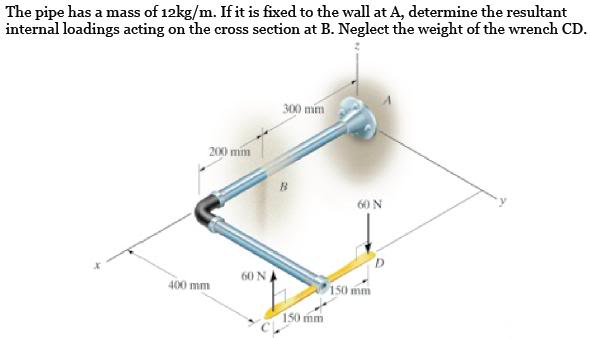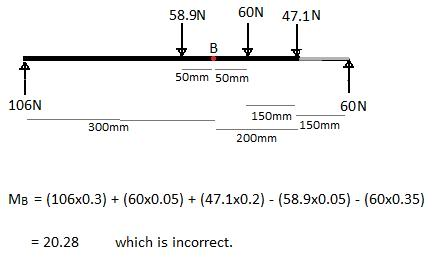# Mechanics of Materials - Internal Moment

• mwmey1

## Homework Statement

Hi everyone. I am attempting to calculate the internal moment at point B about the y-axis. I have correctly found everything else.My calculations:
Weight of 500mm section of pipe = 58.9N
Weight of 400mm section of pipe = 47.1N
Vertical reaction at A = 58.9 + 47.1 = 106N

## Homework Equations

Moment = Force x Distance

## The Attempt at a SolutionI'm not really sure I can do that with the two 60N forces, but I also can't see why not.
The correct answer is 6.23Nm according to the solutions provided.

Any help would be greatly appreciated!
Matt

For the portion of the tube along the X axis due to the tube weight

Moment at B = (1/2)*g*12*(0.2)^2 = 2.35 Nm

For the downward 60N force

Moment at B = 0.05*60 = 3.0 Nm

For the rod portion along the Y axis

Moment at B = 0.2*0.4*g*12 = 9.41 Nm

For the upward 60N force

Moment at B = -0.35*60 = -21 Nm

Total moment at B = 2.35+3.0+9.41-21 = -6.24 Nm, where -ve sign CCW direction

Overt - Thankyou very much for the reply, I'll go and work through your solution now. It seemed like a pretty straight forward problem so it's been annoying me all day!

Much appreciated,
Matt

What does "-ve" in post 2 mean? Where is its definition? Could not find it in an on-line dictionary. Could you point me to an on-line source that defines it?

-'ve = negative, + 'ive = positive , its shorthand notation